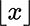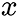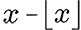# Fract Node

Access: Nodes > Math > FractThe Fract node calculates the fractional part (frac) of an input value by subtracting the floorof the input value from the input value. The Fract node then outputs the result which is only the decimal place remainder of the input.

 Input Output 5.385, 0.164, 3.065, 1.0 0.385, 0.164, 0.065, 0.0 267.19, 105.096, 25.483, 1.0 0.19, 0.096, 0.483, 0.0 3.0, 7.0, 4.0, 1.0 0.0, 0.0, 0.0, 0.0

Note:  For more information on the frac calculation, see here for an explanation provided by MathWorks. For information about the floorcalculation, see Floor node.

The Fract node can be used in multiple ways, one workflow is to use the node to determine where a certain UV coordinate is from within a patch. Using a Fract node after a UV will output just its position within the patch.

Another workflow is to use the Fract node to repeat a part of your texture that's looked up by UV coordinates across each patch. For example, using a Fract node before a Gradient node to repeat the gradient across each patch.

# Fract Node Inputs

 Input The input value of which the fractional part is calculated.# Fract Node Outputs

 Output The decimal remainder of the number only.# Fract Node Properties

No node properties.